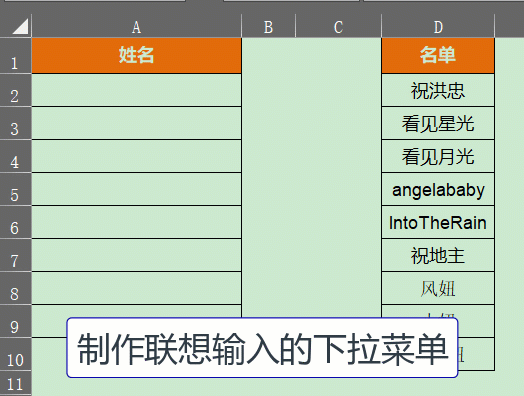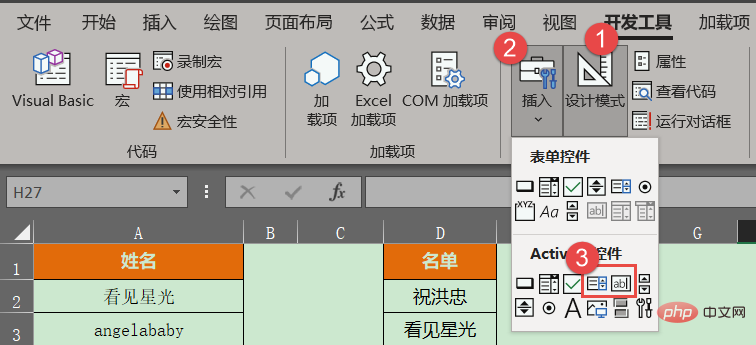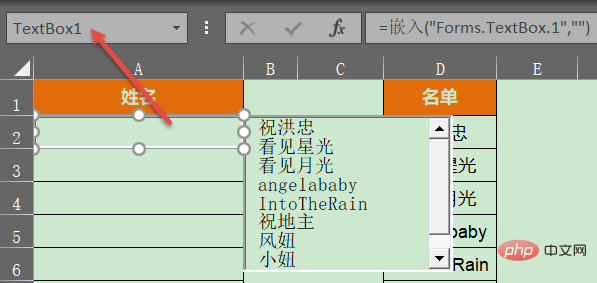# 手把手教你Excel制作动态模糊匹配的下拉菜单EXCEL手机版（内含百种各行模版）：点击查看## 1 丨 制作步骤```'设置文本框和列表框的大小及位置
Private Sub Worksheet_SelectionChange(ByVal Target As Range)
Dim b As Boolean, arr
If Target.Column <> 1 Or Target.Row < 2 Then b = True '如果用户选择的单元格不是第1列或者属于第1行
If Target.Columns.Count > 1 Or Target.Rows.Count > 1 Then b = True '如果用户选择的单元格数量大于1
If b Then
ListBox1.Visible = False '不可见
TextBox1.Visible = False '不可见
Exit Sub '退出程序
End If
With Worksheets("示例") '下拉列表来源内容的所在工作表
arr = .Range("d2:d" & .Cells(Rows.Count, "d").End(3).Row) '数据源
End With
With TextBox1
.Value = ""
.Visible = True '可见
.Top = Target.Top '文本框顶部位置
.Left = Target.Left '文本框左侧位置
.Height = Target.Height '文本框高度
.Width = Target.Width '文本框宽度
.Activate '激活文本框
End With
With ListBox1
.Visible = True '可
.Top = Target.Offset(0, 1).Top
.Left = Target.Offset(0, 1).Left
.Height = Target.Height * 5
.Width = Target.Width
.List = arr '写入数据源数据
End With
End Sub
'根据文本框的输入值动态匹配数据
Private Sub TextBox1_Change()
Dim arr, brr, i&, k&
With Worksheets("示例") '下拉列表来源内容的所在工作表
arr = .Range("d2:d" & .Cells(Rows.Count, "d").End(3).Row) '数据源
End With
If TextBox1.Text = "" Then ListBox1.List = arr: Exit Sub
ReDim brr(1 To UBound(arr))
For i = 1 To UBound(arr)
If InStr(1, arr(i, 1), TextBox1.Text, vbTextCompare) Then  '忽略字母大小写
k = k + 1
brr(k) = arr(i, 1)
End If
Next
ListBox1.List = brr '写入匹配后的数据
End Sub
'如果双击列表框的内容则写入活动单元格
Private Sub ListBox1_DblClick(ByVal Cancel As MSForms.ReturnBoolean)
ActiveCell = ListBox1.Text
With ListBox1
.Clear '清空列表框
.Visible = False
End With
With TextBox1
.Value = ""
.Visible = False
End With
End Sub```

……

## 2 丨 其它

```With Worksheets("示例") '下拉列表来源内容的所在工作表
arr = .Range("d2:d" & .Cells(Rows.Count, "d").End(3).Row) '数据源
End With```1/1